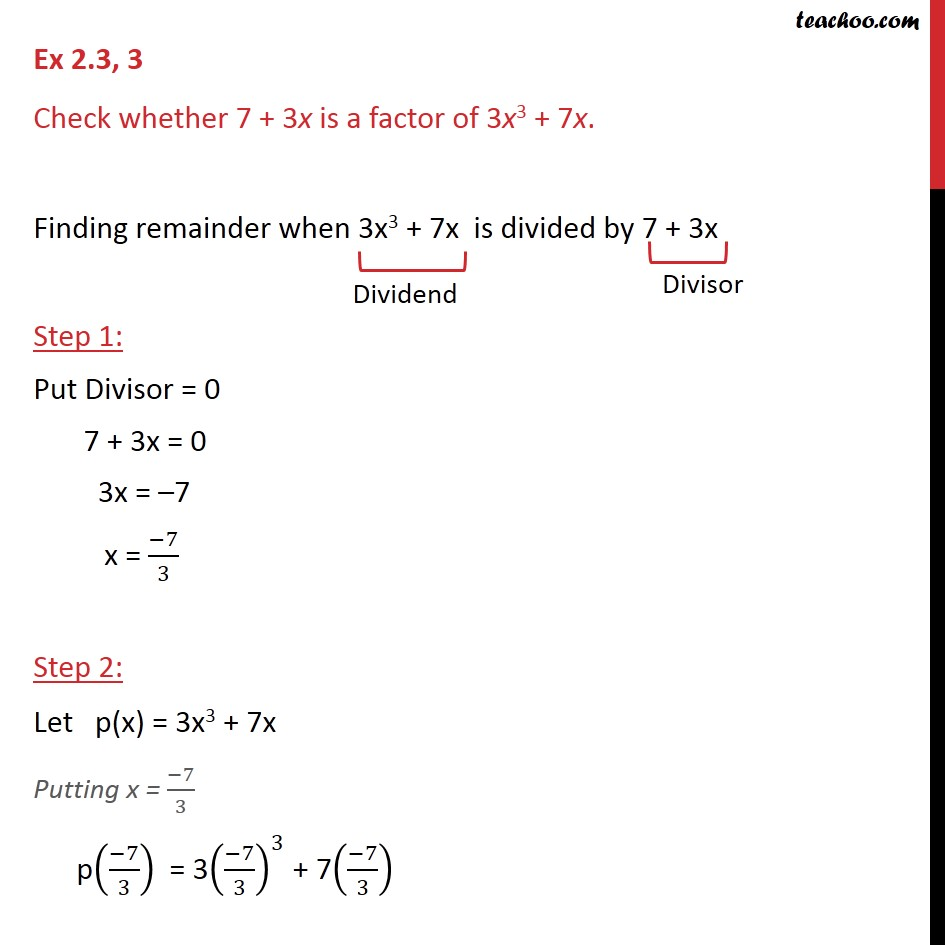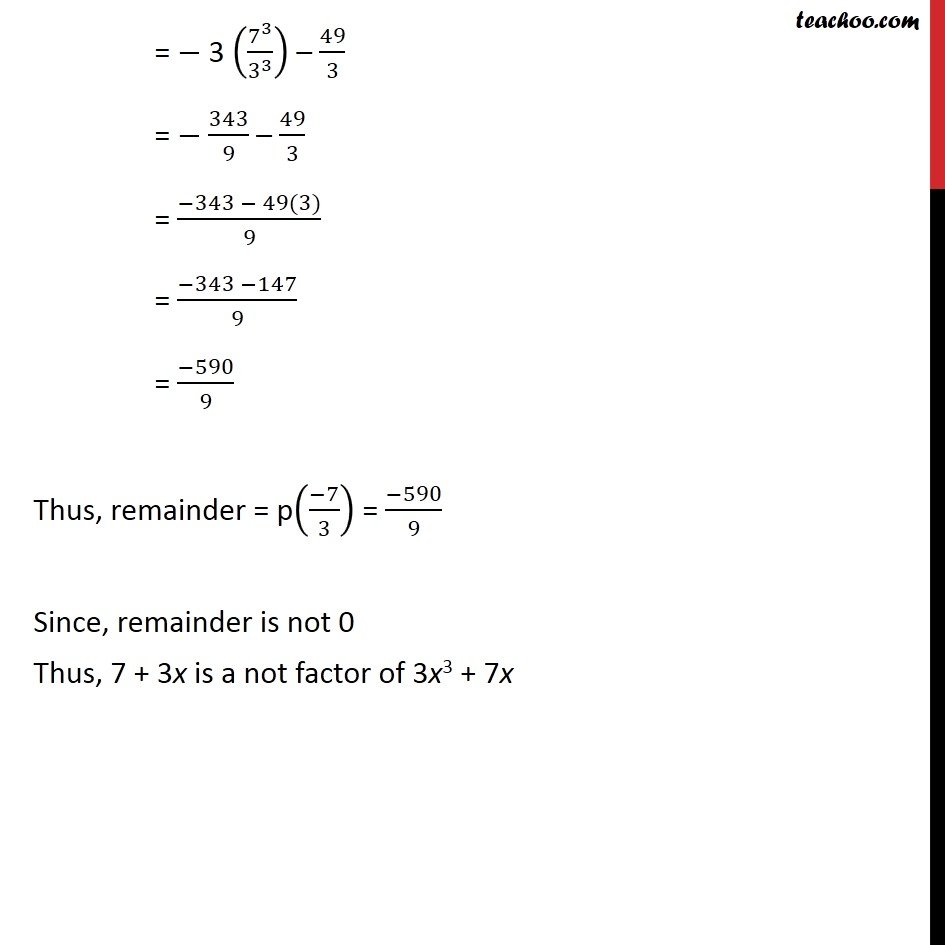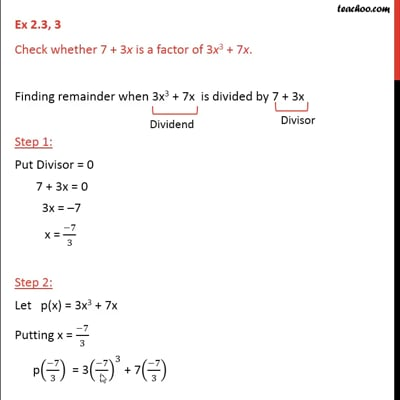Ex 2.3

Chapter 2 Class 9 Polynomials
Serial order wiseThis video is only available for Teachoo black users

Introducing your new favourite teacher - Teachoo Black, at only ₹83 per month

### Transcript

Ex 2.3, 3 Check whether 7 + 3x is a factor of 3x3 + 7x. Finding remainder when 3x3 + 7x is divided by 7 + 3x Step 1: Put Divisor = 0 7 + 3x = 0 3x = 7 x = ( 7)/3 Step 2: Let p(x) = 3x3 + 7x Putting x = ( 7)/3 p(( 7)/3) = 3(( 7)/3)^3 + 7(( 7)/3) = 3 (7^3/3^3 ) 49/3 = 243/9 49/3 = ( 243 49(3))/9 = ( 243 147)/9 = ( 490)/9 Thus, remainder = p(( 7)/3) = ( 490)/9 Since, remainder is not 0 Thus, 7 + 3x is a not factor of 3x3 + 7x